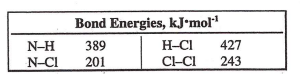# Problem: Calculate ΔH for this gas phase reaction.NH3 (g) + Cl2 (g) → NH2Cl (g) + HCl (g)a) -632 kJb) -188 kJc) +4 kJd) +431 kJ

###### FREE Expert Solution
85% (178 ratings)
###### Problem Details

Calculate Δfor this gas phase reaction.

NH(g) + Cl(g) → NH2Cl (g) + HCl (g)

a) -632 kJ

b) -188 kJ

c) +4 kJ

d) +431 kJWhat scientific concept do you need to know in order to solve this problem?

Our tutors have indicated that to solve this problem you will need to apply the Bond Energy concept. You can view video lessons to learn Bond Energy. Or if you need more Bond Energy practice, you can also practice Bond Energy practice problems.

What is the difficulty of this problem?

Our tutors rated the difficulty ofCalculate ΔH for this gas phase reaction.NH3 (g) + Cl2 (g) →...as medium difficulty.

How long does this problem take to solve?

Our expert Chemistry tutor, Sabrina took 8 minutes and 9 seconds to solve this problem. You can follow their steps in the video explanation above.

What professor is this problem relevant for?

Based on our data, we think this problem is relevant for Professor Del Negro's class at NCSU.